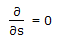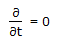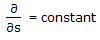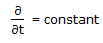# Chemical Engineering - Fluid Mechanics

21.

Steady fluid flow occurs, when the derivative of flow variables satisfy the following condition.

 A.B.C.D.Explanation:

No answer description available for this question. Let us discuss.

22.

What is the maximum theoretical suction lift (metres) of a reciprocating pump ?

 A. 5 B. 10 C. 50 D. 100

Explanation:

No answer description available for this question. Let us discuss.

23.

The terminal velocity of a particle moving through a fluid varies as dpn. The value of n is equal to __________ in Stoke's law regime.

 A. 1 B. 0.5 C. 2 D. 1.5

Explanation:

No answer description available for this question. Let us discuss.

24.

Function of air vessel provided in a reciprocating pump is to

 A. reduce discharge fluctuation. B. reduce the danger of cavitation. C. avoid the necessity of priming. D. increase the pump efficiency.

Explanation:

No answer description available for this question. Let us discuss.

25.

The Prandtl pitot tube measures the

 A. velocity at a point in the flow. B. pressure at a point. C. average flow velocity. D. pressure difference in pipe flow.